# DAV Class 7 Maths Chapter 3 Worksheet 2 Solutions

The DAV Class 7 Maths Book Solutions Pdf and DAV Class 7 Maths Chapter 3 Worksheet 2 Solutions of Rational Numbers as Decimals offer comprehensive answers to textbook questions.

## DAV Class 7 Maths Ch 3 WS 2 Solutions

Question 1.
Express the following rational numbers as decimals by using long division method.
(i) $$\frac{21}{16}$$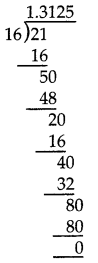Hence $$\frac{21}{16}$$ = 1.3125 (Terminating decimal)

(ii) $$\frac{129}{25}$$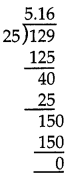Hence $$\frac{129}{25}$$ = 5.16 (Terminating decimal)

(iii) $$\frac{17}{200}$$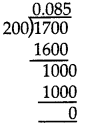Hence $$\frac{17}{200}$$ = 0.085 (Terminating decimal)

(iv) $$\frac{5}{11}$$Hence $$\frac{5}{11}$$ = 0.454545…. = 0.$$\overline{45}$$
(Non – terminating repeating decimal)

(v) $$\frac{22}{7}$$Hence $$\frac{22}{7}$$ = 3.1428 (Non – terminating decimal)

(vi) $$\frac{31}{27}$$Hence $$\frac{31}{27}$$ = 1.148148……..
= 1.$$\overline{148}$$ (Non – terminating and repeating decimal)

(vii) $$\frac{2}{15}$$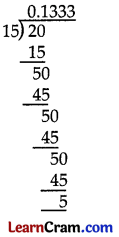Hence $$\frac{2}{15}$$ = 0.1333……….
= 0.13̄ (Non – terminating repeating decimal)

(viii) $$\frac{63}{55}$$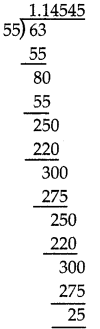Hence $$\frac{63}{55}$$ = 1.14545……….
= 1.1$$\overline{45}$$ (Non – terminating repeating decimal)Question 2.
Without actual division, determine which of the following rational numbers have a terminating decimal representation and which have a non-terminating decimal representation.
(i) $$\frac{11}{4}$$
Prime factors of 4 = 2 × 2
The denominator 7 has no prime factors 22
∴ $$\frac{11}{4}$$ represents a terminating decimal.

(ii) $$\frac{13}{80}$$
Prime factors of 80 = 2 × 2 × 2 × 2 × 5
= 24 × 51
Hence $$\frac{13}{80}$$ represents a terminating decimal.

(iii) $$\frac{15}{11}$$
The denominator 11 has no prime factors as 2m × 5n
Hence $$\frac{15}{11}$$ represents a non-terminating decimal.

(iv) $$\frac{22}{7}$$
The denominator 11 has no prime factors as 2m × 5n
Hence $$\frac{22}{7}$$ represents a non-terminating decimal.

(v) $$\frac{29}{250}$$
Prime factors of 250 = 2 × 5 × 5 × 5 = 21 × 53
Here, the prime factors of 250 has 2 and 5 only
Hence $$\frac{29}{250}$$ represents a terminating decimal.

(vi) $$\frac{37}{21}$$
Prime factors of 21 = 3 × 7
The denominator has no prime factors as 2m × 5n
Hence $$\frac{37}{21}$$ represents a non-terminating decimal.

(vii) $$\frac{49}{14}$$
Prime factors of denominator are in the form 21 × 50
Hence $$\frac{49}{14}$$ represents a terminating decimal

(viii) $$\frac{126}{45}$$
Prime factors of denominator are in the form 20 × 51
Hence $$\frac{126}{45}$$ represents a terminating decimal.

Question 3.
Find the decimal representation of the following rational numbers.
(i) $$\frac{-27}{4}$$
$$\frac{-27}{4}=\frac{-27 \times 25}{4 \times 25}$$
= $$\frac{-675}{100}$$
= -6.75

(ii) $$\frac{-37}{60}$$
60 = 2 × 2 × 3 × 5 (not in the form of 2m × 5n)
So it is a non-terminating decimal.
∴ We will use long division method.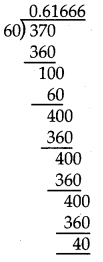Hence $$\frac{-37}{60}$$ = -0.61666…..
= -0.616̄ (Non – terminating repeating decimal)

(iii) $$\frac{-18}{125}$$
125 = 5 × 5 × 5 = 53 × 20 (Terminating decimal)
∴ $$\frac{-18 \times 8}{125 \times 8}=\frac{-144}{1000}$$
= -0.1444

(iv) $$\frac{-15}{8}$$
8 = 2 × 2 × 2 = 23 × 50 (Terminating decimal)
= $$\frac{-15}{8}=\frac{-15 \times 125}{8 \times 125}$$
= $$\frac{-1875}{1000}$$ = -1.875Question 4.
The denominators are 2, 3, 4, 5. They are not all in the form of 2m × 5n.
Therefore, the given numbers is non-terminating decimal representation.

Question 5.
Justify the following statements as true or false.
(i) $$\frac{22}{7}$$ can be represented as terminating decimal.
(F) $$\frac{22}{7}$$, Here prime factors of 7 are not in the form 2m × 5n.
Hence it can not be represented as terminating decimal.

(ii) $$\frac{51}{512}$$ can be represented as a terminating decimal.
(T) $$\frac{51}{512}$$, Here the prime factors of 512 = 2 × 2 × 2 × 2 × 2 × 2 × 2 × 2 × 2 = 29
It is in the form of 2m × 5n i.e. 29 × 5°
Hence it is a terminating decimal representation.

(iii) $$\frac{19}{45}$$ can be represented as a non-terminating repeating decimal.
(iv) $$\frac{3}{17}$$ cannot be represented as a non-terminating repeating decimal.
(v) If $$\frac{3}{2}$$ and $$\frac{7}{5}$$ are terminating decimals, then $$\frac{3}{2}+\frac{7}{5}$$ is also a terminating decimal.
(T) $$\frac{3}{17}$$, Here, prime factors of 17 are not in the form of 2m × 5n. So it is a non-terminating decimal representation.
(vi) If $$\frac{1}{4}$$ and $$\frac{1}{5}$$ both have terminating decimal representation, then $$\frac{1}{4} \times \frac{1}{5}$$ also has a terminating decimal representation.
(T) Here, denominators are 2 and 5, $$\frac{3}{2}+\frac{7}{5}$$ is also a terminating decimal.